## NCERT Solutions for Class 11 Physics Chapter 12 Thermodynamics

These Solutions are part of NCERT Solutions for Class 11 Physics. Here we have given NCERT Solutions for Class 11 Physics Chapter 12 Thermodynamics

Question 1.
A geyser heats water flowing at the rate of 3.0 liters per minute from 27 °C to 77 °C. If the geyser operates on a gas burner, what is the rate of consumption of the fuel if its heat of combustion is 4-0 x 104 J/g ?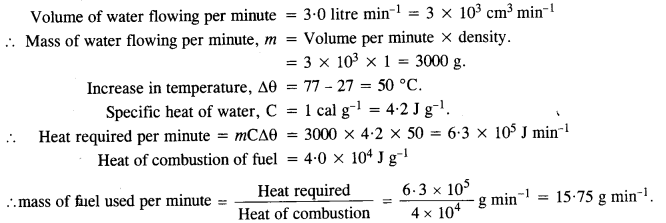Question 2.
What amount of heat must be supplied to 2.0 x 10-2 kg of nitrogen (at room temperature) to raise its temperature by 45°C at constant pressure ? (Molecular) mass of N2 = 28 ; R = 8.3 J mol-1 K-1).
Nitrogen is a diatomic gas. Therefore its molar specific heat at constant pressure isQuestion 3.
Explain why
(a) Two bodies at different temperatures T1 and T2 if brought in thermal contact do not necessarily settle to the mean temperature (T1 + T2)/2.
(b) The coolant in a chemical or a nuclear plant (i.e., the liquid used to prevent the different parts of a plant from getting too hot) should have high specific heat.
(c) Air pressure in a car type increases during driving.
(d) The climate of a harbor town is more temperate than that of a town in a desert at the same latitude.
(a) Two bodies would settle at the mean temperature (T1+ T2)/2 only if they have equal thermal capacities.
(b) A coolant of high specific heat can withdraw more heat out of the chemical or nuclear plant than a coolant of ordinary specific heat.
(c) During driving, the tyres of the car are heated up due to friction between tyres and road. Since heating of tyres causes an increase in temperature, the pressure in the tyres increases (P a T at constant volume).
(d) The relative humidity in a harbour town is more than the relative humidity in a desert town. Therefore, the climatic conditions of a harbour town do not reach extremes of hot or cold making it a temperate zone.

Question 4.
A cylinder with a movable piston contains 3 moles of hydrogen at standard temperature and pressure. The walls of the cylinder are made of a heat insulator, and the piston is insulated by having a pile of sand on it. By what factor does the pressure of the gas increase if the gas is compressed to half its original volume?
Hydrogen is a diatomic gas. Therefore, for hydrogen, ratio of two specific heats is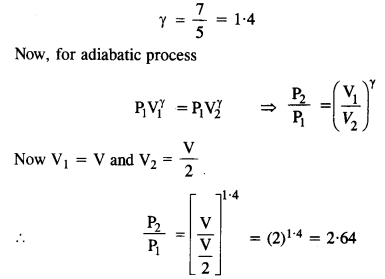Question 5.
In changing the state of a gas adiabatically from an equilibrium state A to another equilibrium state B, an amount of work equal to 22.3 J is done on the system. If the gas is taken form state A to B via a process in which the net heat absorbed by the system is 9.35 cal, how much is the net work done by the system in the latter case ? [Take 1 cal = 4.19 J]
When the state of the gas changes adiabatically from A to B, amount of work done is used to increase the internal energy of the gas.
Increase in internal energy, ΔU = 22.3 J.
In the second case, when state A changes to state B, heat absorbed by the system
ΔQ = 9.35 cal = 9.35 x 4.19J = 39 18 J
Now, using the first law of thermodynamics,
ΔQ =ΔU + ΔW
=> ΔW =ΔQ-ΔU = 39 18-22.3 = 16.88 J

Question 6.
Two cylinders A and B of equal capacity are connected to each other via a stopcock. A contains gas at standard temperature and pressure. B is completely evacuated. The entire system is thermally insulated. The stopcock is suddenly opened. Answer the following :
(a) What is the final pressure of the gas in A and B?
(b) What is the change in the internal energy of the gas?
(c) What is the change in the temperature of the gas?
Do the intermediate states of the system (before settling to the final equilibrium state) lie of its P-V-T surface ?
(a) Since the final temperature and initial temperature remain the same (isothermal process).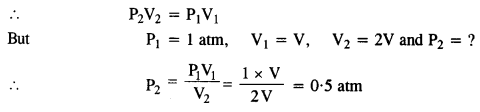(b) Since the temperature of the system remains unchanged, the change in internal energy is zero.

(c) The system being thermally insulated, there is no change in temperature (because of free expansion)

(d) The expansion is a free expansion. Therefore, the intermediate states are non-equilibrium states and the gas equation is not satisfied in these states. As a result, the gas can not return to an equilibrium state which lie on the P-V-T surface.

Question 7.
A steam engine delivers 5.4 x 108 J of work per minute and services 3.6 x 109 J of heat per minute from its boiler. What is the efficiency of the engine? How much heat is wasted per minute?
Output i.e., useful work done per minute = 5.4 x 108J
Input i.e., heat absorbed per minute = 3.6 X 109 J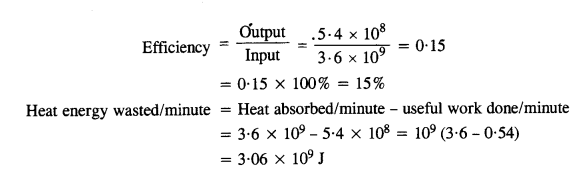Question 8.
An electric heater supplies heat to a system at a rate of 100 W. If the system performs work at a rate of 75 joules per second, at what rate is the internal energy increasing?
Rate of heat supplied to the system is 100 W = 100 Js-1
Rate of work done by the system is 75Js-1.
From the first law of thermodynamics, we have
∆ Q = ∆ U + ∆ W ……..(1)
If the above parameters are observed for a time‘∆ t’,
$$\frac{\Delta \mathrm{Q}}{\Delta \mathrm{t}}=\frac{\Delta \mathrm{U}}{\Delta \mathrm{t}}+\frac{\Delta \mathrm{W}}{\Delta \mathrm{t}}$$ …….(2)
where $$\frac{\Delta Q}{\Delta t}$$ is the rate of heat supplied to the system for the given time, $$\frac{\Delta U}{\Delta t}$$ is the rate of increase in internal energy of the system for the given time and $$\frac{\Delta W}{\Delta t}$$ is the
rate of work done by the system in the given time.
∴ From equation (2), we have
100 = $$\frac{\Delta U}{\Delta t}$$ + 75
∴ $$\frac{\Delta U}{\Delta t}$$ = 25 Js-1
Therefore the internal energy increases at rate of 25 Joules per second.

Question 9.
A thermodynamic system is taken from an original state to an intermediate state by the linear process shown in Fig.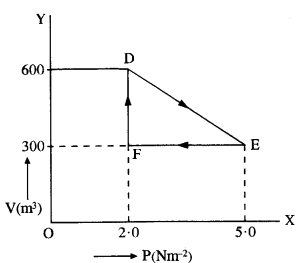Its volume is then reduced to the original value from E to F by an isobaric process. Calculate the work done by the gas from D to E to F.